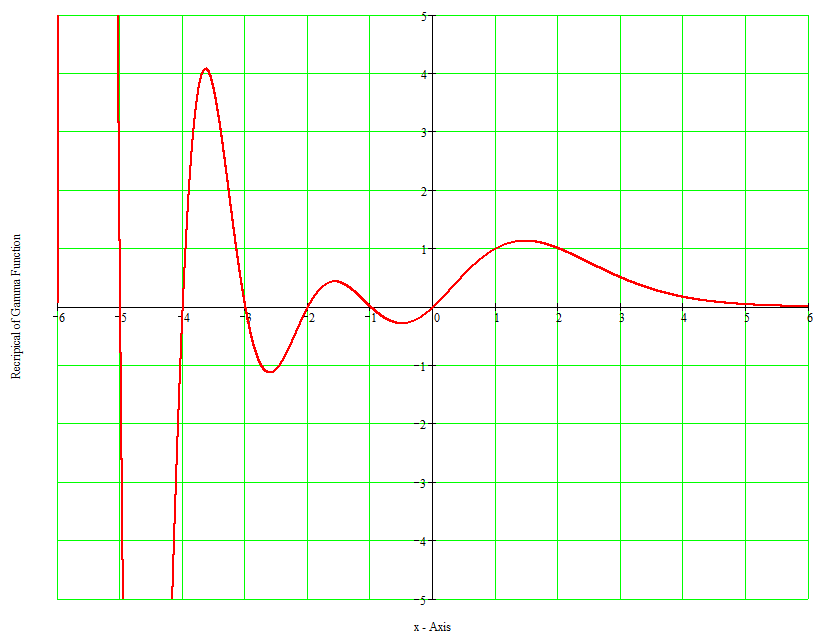# Gamma Function

The Gamma function Gam(x) = (x−1)! = 1*2*3*...*(x−1) if x is an integer > 0. Gamma is defined for all values of x except zero and negative integers.

For example, Gam(5) = 1*2*3*4 = 24.

Here are some notes from my program XPCalc - Extra Precision Floating-Point Calculator http://www.oocities.org/hjsmithh/download.html#XPCalc :

Gam(x) = GammaFunction(x) = (x−1)!:

If |x| > +4.05997,83000,01705,88908,81E+18, an error message is displayed: "Number too large for Gamma function". Otherwise, for x >= −5, the asymptotic formula with Bernoulli numbers is used to compute this. If x is an integer <= zero, an alarm is displayed. For non-integer negative x < −5, the reflection formula is used: Gam(x) = Pi / (Gam(y) * Sin((Pi * y)), where y = 1 − x. For large x (x > n),

Ln(Gam(x)) =~ (x − 0.5)*Ln(x) − x + Ln(2*Pi)/2 + Sum{k=1,2,...}[B(2*k) / (2*k*(2*k−1)*x^(2*k−1))],

where B(2*k) are Bernoulli numbers. For small x,

Gam(x) = Gam(n + x) / x / (x+1) / (x+2) / ... / (n−1+x).

This is based on Gam(x) = Gam(x+1) / x. n is computed by a heuristic, n = (digits^1.5) / 13.0 + 1, where digits is the current decimal digits in a computed mantissa. Actually for very small integer n greater than 0, depending on the precision desired, the factorial function is used: Gam(n) = (n−1)! = 1*2*3*4*...*(n−1).

There are times when you are computing the factorial function Fac(n) = n! that it is best to use the Gamma function. For very large n, depending on the precision desired, use Gam(n+1) to compute n!.See: Gamma Function -- From MathWorld
And: Wolfram Function Evaluation -- Gamma

Also: Factorial -- From MathWorld
And: Wolfram Function Evaluation -- Factorial

This page accessedtimes since April 5, 2005.
Page created by: hjsmithh@sbcglobal.net
Changes last made on Wednesday, 25-Mar-09 09:52:43 PDT